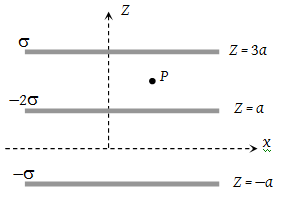Three infinitely long charge sheets are placed as shown in figure. The electric field at point P is(1) $\frac{2\sigma }{{\epsilon }_{o}}\stackrel{^}{k}$

(2) $-\frac{2\sigma }{{\epsilon }_{o}}\stackrel{^}{k}$

(3) $\frac{4\sigma }{{\epsilon }_{o}}\stackrel{^}{k}$

(4) $-\frac{4\sigma }{{\epsilon }_{o}}\stackrel{^}{k}$